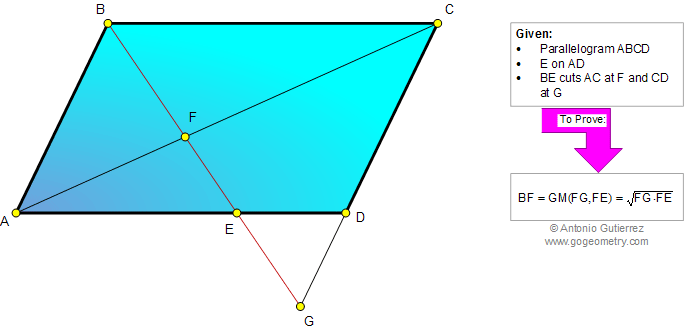# Online Geometry Problem 834: Parallelogram, Diagonal, Similarity, Geometric Mean, Mean Proportional. Level: High School, SAT Prep, College, Mathematics Education

 The figure below shows a parallelogram ABCD. E is a point on AD, BE cuts diagonal AC at F and CD at G. Prove that BF is the geometric mean or mean proportional of FG and FE.See also: Typography of problem 834.
 Home | Search | Geometry | Problems | All Problems | Open Problems | Visual Index | 10 Problems | 831-840 | Triangles | Parallelogram | Similarity, Ratios, Proportions | Geometric Mean | Email | Solution / comment | by Antonio Gutierrez